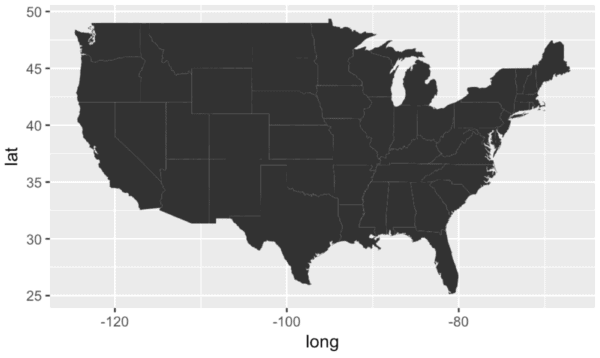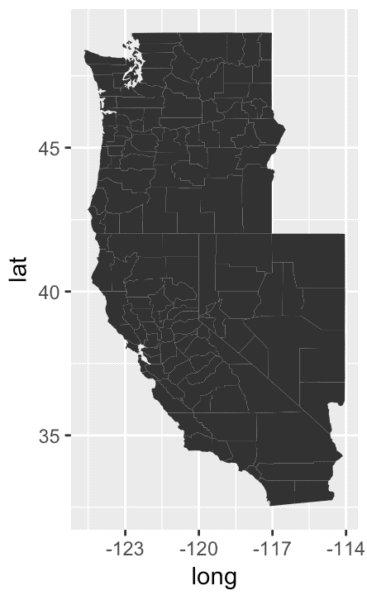Want to share your content on R-bloggers? click here if you have a blog, or here if you don't.

To master data science, you need to practice.

This sounds easy enough, but in reality, many people have no idea how to practice.

A full explanation of how to practice is beyond the scope of this blog post, but I can give you a quick tip here:

You need to master the most important techniques, and then practice those techniques in small scripts until you know them backwards and forwards.

Your goal should be to be able to write code “with your eyes closed.”

With that in mind, I want to give you a few small scripts that you can practice. Here are a few small scripts to create a set of maps. (As you examine these, pay close attention to how simple they are. How many functions and commands do you actually need to know?)

Let’s start with a simple one: create a map of the United States.

```# INSTALL PACKAGE: tidyverse
library(tidyverse)

# MAP USA
map_data("usa") %>%
ggplot(aes(x = long, y = lat, group = group)) +
geom_polygon()

```Three lines of code (four, if you count the library() function).

That’s it. Three lines to make a map of the US.

And if you change just 5 characters (change usa to state) you can generate a map of the states of the United States:

```# MAP USA (STATES)
map_data("state") %>%
ggplot(aes(x = long, y = lat, group = group)) +
geom_polygon()
```If you add an additional line, you can use filter to subset your data and map only a few states.

```# MAP CALIFORNIA, NEVADA, OREGON, WASHINGTON
# - to do this, we're using dplyr::filter()
#   ... otherwise, it's almost exactly the same as the previous code
map_data("state") %>%
ggplot(aes(x = long, y = lat, group = group)) +
geom_polygon()
```What I want to emphasize is how easy this is if you just know how filter works and how the pipe operator (%>%) works.

If you make another simple change, you can create a county level map of those states:

```# MAP CALIFORNIA, NEVADA, OREGON, WASHINGTON
#  (Counties)

map_data("county") %>%
ggplot(aes(x = long, y = lat, group = group)) +
geom_polygon()
```Finally, a few more changes can get you a map of the world:

```# MAP WORLD

map_data("world") %>%
ggplot(aes(x = long, y = lat, group = group)) +
geom_polygon()
```… and then a map of a single country, like Japan:

```# MAP JAPAN

map_data("world") %>%
filter(region == 'Japan') %>%
ggplot(aes(x = long, y = lat, group = group)) +
geom_polygon()
```I want to point out again that all of these maps were created with very simple variations on our original 3 line script.

It really doesn’t take that much to achieve competence in creating basic maps like this.

# Practice small to learn big

You might be asking: why bother practicing these? They’re not terribly useful.

You need to understand that large projects are built from dozens of small snippets of code like these.

Moreover, these little snippets of code are made up of a small set of functions that you can break down and learn one command at a time.

So the path to mastery involves first mastering syntax of small individual commands and functions. After you’ve memorized the syntax of individual functions and commands, little scripts like this give you an opportunity to put those commands together into something a little more complex.

Later, these little scripts can be put together with other code snippets to perform more complicated analyses.

If you can practice (and master) enough small scripts like this, it becomes dramatically easier to execute larger projects quickly and confidently.

For example, by mastering the little scripts above, you put yourself on a path to creating something more like this:If you want to achieve real fluency, you need to practice small before you practice big. So find little scripts like this, break them down, and drill them until you can type them without hesitation. You’ll thank me later.

(You’re welcome.)

# A quick exercise for you

Count up the commands and arguments that we used in the little scripts above.

For the sake of simplicity, don’t count the data features that you might need (like the “region” column, etc), just count the number of functions, commands, and arguments that you need to know.

How many? If you really, really pushed yourself, how long would it take to memorize those commands?

# Sign up now, and discover how to rapidly master data science

To rapidly master data science, you need a plan.

You need to be highly systematic.

Sign up for our email list right now, and you’ll get our “Data Science Crash Course.”

In it you’ll discover:

• A step-by-step learning plan
• How to create the essential data visualizations
• How to perform the essential data wrangling techniques
• How to get started with machine learning
• How much math you need to learn (and when to learn it)
• And more …